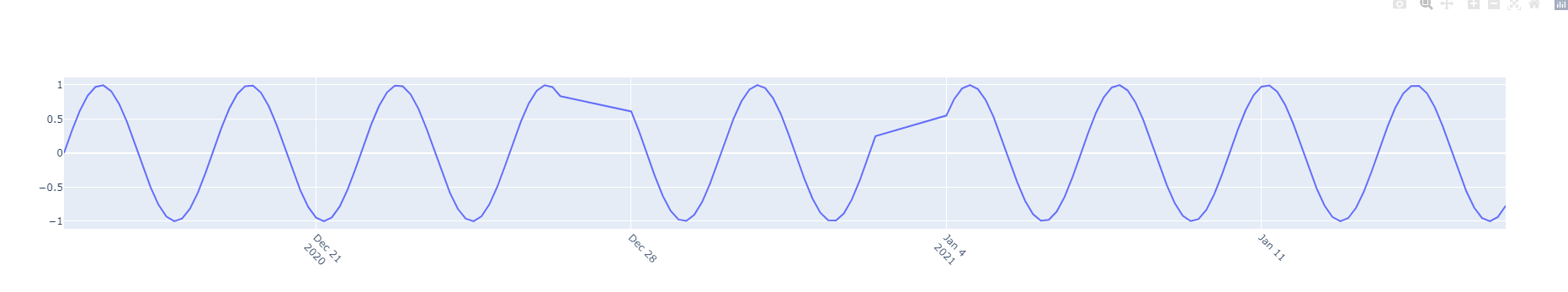# rangebreaks for datetime axises, the value+dvalue doesn't work with bounds

It looks that the `value+dvalue` mode doesn’t work well with the `bounds` mode for `rangebreak` parameter of the datetime axis. Below is an simple example to replicate the issue:

#### packages import and data preparation:

import numpy as np import pandas as pd from pandas.tseries.offsets import CustomBusinessHour, BusinessDay from pandas.tseries.holiday import USFederalHolidayCalendar import plotly.offline as py import plotly.graph_objs as go x = pd.bdate_range(‘2020-12-15’, ‘2021-01-15’, freq=CustomBusinessHour(calendar=USFederalHolidayCalendar())) y = np.sin(np.arange(len(x))/3)

#### Scenario I, bounds only to exclude non-business hours and weekends

layout1=go.Layout(xaxis=dict(tickangle=45, rangebreaks=[ dict(pattern=‘hour’, bounds=[17, 9]), dict(bounds=[‘sat’, ‘mon’]) ])) fig1 = go.Figure(data=go.Scatter(x=x, y=y), layout=layout1) fig1.show()We see the discontinuity on business-day holidays 2020-12-25, 2021-01-01 as expected.

#### Scenario II, trying to exclude the holidays with `values` dict

missing_date2 = list(set(pd.bdate_range(‘2020-12-15’, ‘2021-01-15’)) - set(x.normalize())) layout2=go.Layout(xaxis=dict(tickangle=45, rangebreaks=[ dict(values=missing_date2), dict(pattern=‘hour’, bounds=[17, 9]), dict(bounds=[‘sat’, ‘mon’]), ])) fig2 = go.Figure(data=go.Scatter(x=x, y=y), layout=layout2) fig2.show()

#### Scenario III, use `values` for day level only:

Even I don’t use `bounds` for weekends, it still faces same problem:

missing_date3 = list(set(pd.date_range(‘2020-12-15’, ‘2021-01-15’)) - set(x.normalize())) layout3=go.Layout(xaxis=dict(tickangle=45, rangebreaks=[ dict(values=missing_date3), dict(pattern=‘hour’, bounds=[17, 9]), ])) fig3 = go.Figure(data=go.Scatter(x=x, y=y), layout=layout3) fig3.show()Can someone help take a look please?